# Wrap-Up

### Answers to Self Review Exercises

4.1

a) sequence, selection, repetition. b) if...else. c) counter-controlled (or definite). d) sentinel, signal, flag or dummy. e) sequence. f) 0 (zero). g) strongly typed. h) prefixed.

4.2

a) True. b) False. A set of statements contained within a pair of braces ({ and }) is called a block. c) False. A repetition statement specifies that an action is to be repeated while some condition remains true. d) True. e) True. f) False. The primitive types (boolean, char, byte, short, int, long, float and double) are portable across all computer platforms that support Java. g) True. h) False. The unary cast operator (double) creates a temporary floating-point copy of its operand. i) False. Instance variables of type boolean are given the value false by default. j) True.

4.3
```x = x + 1;
x += 1;
++x;
x++;
```
4.4
1. z = x++ + y;
2. if ( count > 10 ) System.out.println( "Count is greater than 10" );
3. total -= --x;
4. q %= divisor; q = q % divisor;
4.5
1. int sum, x;
2. x = 1;
3. sum = 0;
4. sum += x; or sum = sum + x;
5. System.out.printf( "The sum is: %d ", sum );
4.6

The program is as follows:

``` 1 // Calculate the sum of the integers from 1 to 10
2 public class Calculate
3 {
4 public static void main( String args[] )
5 {
6 int sum;
7 int x;
8
```
9 x = 1; // initialize x to 1 for counting 10 sum = 0; // initialize sum to 0 for totaling 11 12 while ( x <= 10 ) // while x is less than or equal to 10 13 { 14 sum += x; // add x to sum 15 ++x; // increment x 16 } // end while 17 18 System.out.printf( "The sum is: %d ", sum ); 19 } // end main 20 21 } // end class Calculate
 ```The sum is: 55 ```
4.7

product = 25, x = 6

4.8
1. Error: The closing right brace of the while statement's body is missing.

Correction: Add a closing right brace after the statement ++c;.

2. Error: The semicolon after else results in a logic error. The second output statement will always be executed.

Correction: Remove the semicolon after else.

4.9

The value of the variable z is never changed in the while statement. Therefore, if the loop-continuation condition ( z >= 0 ) is true, an infinite loop is created. To prevent an infinite loop from occurring, z must be decremented so that it eventually becomes less than 0.

### Exercises

4.10

Compare and contrast the if single-selection statement and the while repetition statement. How are these two statements similar? How are they different?

4.11

Explain what happens when a Java program attempts to divide one integer by another. What happens to the fractional part of the calculation? How can a programmer avoid that outcome?

4.12

Describe the two ways in which control statements can be combined.

4.13

What type of repetition would be appropriate for calculating the sum of the first 100 positive integers? What type of repetition would be appropriate for calculating the sum of an arbitrary number of positive integers? Briefly describe how each of these tasks could be performed.

4.14

What is the difference between preincrementing a variable and postincrementing a variable?

4.15

Identify and correct the errors in each of the following pieces of code. [Note: There may be more than one error in each piece of code.]

1. if ( age >= 65 ); System.out.println( "Age greater than or equal to 65" ); else System.out.println( "Age is less than 65 )";
2. int x = 1, total; while ( x <= 10 ) { total += x; ++x; }
3. while ( x <= 100 ) total += x; ++x;
4. while ( y > 0 ) { System.out.println( y ); ++y;
4.16

What does the following program print?

``` 1 public class Mystery
2 {
3 public static void main( String args[] )
4 {
5 int y;
6 int x = 1;
7 int total = 0;
8
9 while ( x <= 10 )
10 {
11 y = x * x;
12 System.out.println( y );
13 total += y;
14 ++x;
15 } // end while
16
17 System.out.printf( "Total is %d
", total );
18 } // end main
19
20 } // end class Mystery
```

For Exercise 4.17 through Exercise 4.20, perform each of the following steps:

2. Formulate the algorithm using pseudocode and top-down, stepwise refinement.

3. Write a Java program.

4. Test, debug and execute the Java program.

5. Process three complete sets of data.

4.17

Drivers are concerned with the mileage their automobiles get. One driver has kept track of several tankfuls of gasoline by recording the miles driven and gallons used for each tankful. Develop a Java application that will input the miles driven and gallons used (both as integers) for each tankful. The program should calculate and display the miles per gallon obtained for each tankful and print the combined miles per gallon obtained for all tankfuls up to this point. All averaging calculations should produce floating-point results. Use class Scanner and sentinel-controlled repetition to obtain the data from the user.

4.18

Develop a Java application that will determine whether any of several department-store customers has exceeded the credit limit on a charge account. For each customer, the following facts are available:

1. account number
2. balance at the beginning of the month
3. total of all items charged by the customer this month
4. total of all credits applied to the customer's account this month
5. allowed credit limit.

The program should input all these facts as integers, calculate the new balance (= beginning balance + charges credits), display the new balance and determine whether the new balance exceeds the customer's credit limit. For those customers whose credit limit is exceeded, the program should display the message "Credit limit exceeded".

4.19

A large company pays its salespeople on a commission basis. The salespeople receive \$200 per week plus 9% of their gross sales for that week. For example, a salesperson who sells \$5,000 worth of merchandise in a week receives \$200 plus 9% of \$5,000, or a total of \$650. You have been supplied with a list of the items sold by each salesperson. The values of these items are as follows:

 Item Value 1 239.99 2 129.75 3 99.95 4 350.89

Develop a Java application that inputs one salesperson's items sold for last week and calculates and displays that salesperson's earnings. There is no limit to the number of items that can be sold by a salesperson.

4.20

Develop a Java application that will determine the gross pay for each of three employees. The company pays straight time for the first 40 hours worked by each employee and time and a half for all hours worked in excess of 40 hours. You are given a list of the employees of the company, the number of hours each employee worked last week and the hourly rate of each employee. Your program should input this information for each employee and should determine and display the employee's gross pay. Use class Scanner to input the data.

4.21

The process of finding the largest value (i.e., the maximum of a group of values) is used frequently in computer applications. For example, a program that determines the winner of a sales contest would input the number of units sold by each salesperson. The salesperson who sells the most units wins the contest. Write a pseudocode program and then a Java application that inputs a series of 10 integers and determines and prints the largest integer. Your program should use at least the following three variables:

1. counter: A counter to count to 10 (i.e., to keep track of how many numbers have been input and to determine when all 10 numbers have been processed).
2. number: The integer most recently input by the user.
3. largest: The largest number found so far.
4.22

Write a Java application that uses looping to print the following table of values:

 N 10*N 100*N 1000*N 1 10 100 1000 2 20 200 2000 3 30 300 3000 4 40 400 4000 5 50 500 5000
4.23

Using an approach similar to that for Exercise 4.21, find the two largest values of the 10 values entered. [Note : You may input each number only once.]

4.24

Modify the program in Fig. 4.12 to validate its inputs. For any input, if the value entered is other than 1 or 2, keep looping until the user enters a correct value.

4.25

What does the following program print?

``` 1 public class Mystery2
2 {
3 public static void main( String args[] )
4 {
5 int count = 1;
6
7 while ( count <= 10 )
8 {
9 System.out.println( count % 2 == 1 ? "****" : "++++++++" );
10 ++count;
11 } // end while
12 } // end main
13
14 } // end class Mystery2
```
4.26

What does the following program print?

``` 1 public class Mystery3
2 {
3 public static void main( String args[] )
4 {
5 int row = 10;
6 int column;
7
8 while ( row >= 1 )
9 {
10 column = 1;
11
12 while ( column <= 10 )
13 {
14 System.out.print( row % 2 == 1 ? "<" : ">" );
15 ++column;
16 } // end while
17
18 --row;
19 System.out.println();
20 } // end while
21 } // end main
22
23 } // end class Mystery3
```
4.27

(Dangling-else Problem) Determine the output for each of the given sets of code when x is 9 and y is 11 and when x is 11 and y is 9. Note that the compiler ignores the indentation in a Java program. Also, the Java compiler always associates an else with the immediately preceding if unless told to do otherwise by the placement of braces ({}). On first glance, the programmer may not be sure which if an else matchesthis situation is referred to as the "dangling-else problem." We have eliminated the indentation from the following code to make the problem more challenging. [Hint: Apply the indentation conventions you have learned.]

1. if ( x < 10 ) if ( y > 10 ) System.out.println( "*****" ); else System.out.println( "#####" ); System.out.println( "\$\$\$\$\$" );
2. if ( x < 10 ) { if ( y > 10 ) System.out.println( "*****" ); } else { System.out.println( "#####" ); System.out.println( "\$\$\$\$\$" ); }
4.28

(Another Dangling-else Problem) Modify the given code to produce the output shown in each part of the problem. Use proper indentation techniques. Make no changes other than inserting braces and changing the indentation of the code. The compiler ignores indentation in a Java program. We have eliminated the indentation from the given code to make the problem more challenging. [Note : It is possible that no modification is necessary for some of the parts.]

``` if ( y == 8 )
if ( x == 5 )
System.out.println( "@@@@@" );
else
System.out.println( "#####" );
System.out.println( "\$\$\$\$\$" );
System.out.println( "&&&&&" );
```

1. Assuming that x = 5 and y = 8, the following output is produced:

``` @@@@@
\$\$\$\$\$
&&&&&
```
2. Assuming that x = 5 and y = 8, the following output is produced:

``` @@@@@
```
3. Assuming that x = 5 and y = 8, the following output is produced:

``` @@@@@
&&&&&
```
4. Assuming that x = 5 and y = 7, the following output is produced. [Note : The last three output statements after the else are all part of a block.]

``` #####
\$\$\$\$\$
&&&&&
```
4.29

Write an application that prompts the user to enter the size of the side of a square, then displays a hollow square of that size made of asterisks. Your program should work for squares of all side lengths between 1 and 20.

4.30

(Palindromes) A palindrome is a sequence of characters that reads the same backward as forward. For example, each of the following five-digit integers is a palindrome: 12321, 55555, 45554 and 11611. Write an application that reads in a five-digit integer and determines whether it is a palindrome. If the number is not five digits long, display an error message and allow the user to enter a new value.

4.31

Write an application that inputs an integer containing only 0s and 1s (i.e., a binary integer) and prints its decimal equivalent. [Hint: Use the remainder and division operators to pick off the binary number's digits one at a time, from right to left. In the decimal number system, the rightmost digit has a positional value of 1 and the next digit to the left has a positional value of 10, then 100, then 1000, and so on. The decimal number 234 can be interpreted as 4 * 1 + 3 * 10 + 2 * 100. In the binary number system, the rightmost digit has a positional value of 1, the next digit to the left has a positional value of 2, then 4, then 8, and so on. The decimal equivalent of binary 1101 is 1 * 1 + 0 * 2 + 1 * 4 + 1 * 8, or 1 + 0 + 4 + 8 or, 13.]

4.32

Write an application that uses only the output statements

``` System.out.print( "* " );
System.out.print( " " );
System.out.println();
```

to display the checkerboard pattern that follows. Note that a System.out.println method call with no arguments causes the program to output a single newline character. [Hint: Repetition statements are required.]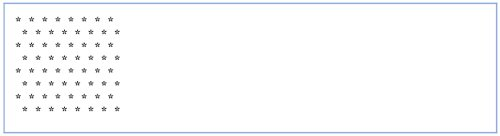4.33

Write an application that keeps displaying in the command window the multiples of the integer 2namely, 2, 4, 8, 16, 32, 64, and so on. Your loop should not terminate (i.e., create an infinite loop). What happens when you run this program?

4.34

What is wrong with the following statement? Provide the correct statement to add one to the sum of x and y.

``` System.out.println( ++(x + y) );
```
4.35

Write an application that reads three nonzero values entered by the user and determines and prints whether they could represent the sides of a triangle.

4.36

Write an application that reads three nonzero integers and determines and prints whether they could represent the sides of a right triangle.

4.37

A company wants to transmit data over the telephone, but is concerned that its phones may be tapped. It has asked you to write a program that will encrypt the data so that it may be transmitted more securely. All the data is transmitted as four-digit integers. Your application should read a four-digit integer entered by the user and encrypt it as follows: Replace each digit with the result of adding 7 to the digit and getting the remainder after dividing the new value by 10. Then swap the first digit with the third, and swap the second digit with the fourth. Then print the encrypted integer. Write a separate application that inputs an encrypted four-digit integer and decrypts it to form the original number.

4.38

The factorial of a nonnegative integer n is written as n! (pronounced "n factorial") and is defined as follows: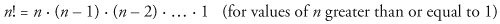and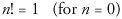For example, 5! = 5 . 4 . 3 . 2 . 1, which is 120.

1. Write an application that reads a nonnegative integer and computes and prints its factorial.
2. Write an application that estimates the value of the mathematical constant e by using the formula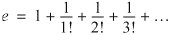3. Write an application that computes the value of ex by using the formula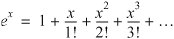Java How to Program (6th Edition) (How to Program (Deitel))
ISBN: 0131483986
EAN: 2147483647
Year: 2003
Pages: 615